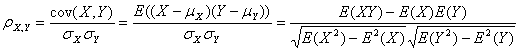# python机器学习案例系列教程——推荐系统

python数据挖掘系列教程

1. 基于用户行为数据的协同过滤算法
2. 基于内容数据的过滤算法

## 基于用户行为数据的算法

1.1 基于用户的协同过滤算法（user-based CF）

1.2 基于商品的协同过滤

1.3 基于模型的协同过滤

## 基于内容数据的推荐

• 利用领域专家给项目打标签的方法 ， 也即传统的分类系统（Taxonomy），
• 另一种是用户给项目打标签， 也即大众分类系统 （Folksolomy）。

## Knn(邻近算法)

K最近邻(k-Nearest Neighbor，KNN)分类算法，是一个理论上比较成熟的方法，也是最简单的机器学习算法之一。该方法的思路是：如果一个样本在特征空间中的k个最相似(即特征空间中最邻近)的样本中的大多数属于某一个类别，则该样本也属于这个类别。KNN算法中，所选择的邻居都是已经正确分类的对象。该方法在定类决策上只依据最邻近的一个或者几个样本的类别来决定待分样本所属的类别。 KNN方法虽然从原理上也依赖于极限定理，但在类别决策时，只与极少量的相邻样本有关。由于KNN方法主要靠周围有限的邻近的样本，而不是靠判别类域的方法来确定所属类别的，因此对于类域的交叉或重叠较多的待分样本集来说，KNN方法较其他方法更为适合

## 基于矩阵分解的推荐算法

${R}_{m×n}\approx {P}_{m×k}×{Q}_{k×n}={\stackrel{^}{R}}_{m×n}$

${e}_{i,j}^{2}={\left({r}_{i,j}-{\stackrel{^}{r}}_{i,j}\right)}^{2}={\left({r}_{i,j}-\sum _{k=1}^{K}{p}_{i,k}{q}_{k,j}\right)}^{2}$

$min\phantom{\rule{thickmathspace}{0ex}}loss=\sum _{{r}_{i,j}\ne -}{e}_{i,j}^{2}$

$\frac{\mathrm{\partial }}{\mathrm{\partial }{p}_{i,k}}{e}_{i,j}^{2}=-2\left({r}_{i,j}-\sum _{k=1}^{K}{p}_{i,k}{q}_{k,j}\right){q}_{k,j}=-2{e}_{i,j}{q}_{k,j}$

$\frac{\mathrm{\partial }}{\mathrm{\partial }{q}_{k,j}}{e}_{i,j}^{2}=-2\left({r}_{i,j}-\sum _{k=1}^{K}{p}_{i,k}{q}_{k,j}\right){p}_{i,k}=-2{e}_{i,j}{p}_{i,k}$

${{p}_{i,k}}^{\prime }={p}_{i,k}-\alpha \frac{\mathrm{\partial }}{\mathrm{\partial }{p}_{i,k}}{e}_{i,j}^{2}={p}_{i,k}+2\alpha {e}_{i,j}{q}_{k,j}$

${{q}_{k,j}}^{\prime }={q}_{k,j}-\alpha \frac{\mathrm{\partial }}{\mathrm{\partial }{q}_{k,j}}{e}_{i,j}^{2}={q}_{k,j}+2\alpha {e}_{i,j}{p}_{i,k}$

${E}_{i,j}^{2}={\left({r}_{i,j}-\sum _{k=1}^{K}{p}_{i,k}{q}_{k,j}\right)}^{2}+\frac{\beta }{2}\sum _{k=1}^{K}\left({p}_{i,k}^{2}+{q}_{k,j}^{2}\right)$

$\frac{\mathrm{\partial }}{\mathrm{\partial }{p}_{i,k}}{E}_{i,j}^{2}=-2\left({r}_{i,j}-\sum _{k=1}^{K}{p}_{i,k}{q}_{k,j}\right){q}_{k,j}+\beta {p}_{i,k}=-2{e}_{i,j}{q}_{k,j}+\beta {p}_{i,k}$

$\frac{\mathrm{\partial }}{\mathrm{\partial }{q}_{k,j}}{E}_{i,j}^{2}=-2\left({r}_{i,j}-\sum _{k=1}^{K}{p}_{i,k}{q}_{k,j}\right){p}_{i,k}+\beta {q}_{k,j}=-2{e}_{i,j}{p}_{i,k}+\beta {q}_{k,j}$

${{p}_{i,k}}^{\prime }={p}_{i,k}-\alpha \left(\frac{\mathrm{\partial }}{\mathrm{\partial }{p}_{i,k}}{e}_{i,j}^{2}+\beta {p}_{i,k}\right)={p}_{i,k}+\alpha \left(2{e}_{i,j}{q}_{k,j}-\beta {p}_{i,k}\right)$

${{q}_{k,j}}^{\prime }={q}_{k,j}-\alpha \left(\frac{\mathrm{\partial }}{\mathrm{\partial }{q}_{k,j}}{e}_{i,j}^{2}+\beta {q}_{k,j}\right)={q}_{k,j}+\alpha \left(2{e}_{i,j}{p}_{i,k}-\beta {q}_{k,j}\right)$

$\sum _{k=1}^{K}{p}_{i,k}{q}_{k,j}$

## Svd(奇异值分解)

svd的全称是：Singular Value Decomposition，翻译过来是奇异值分解，是一种矩阵分解的方法。其实，这个方法是提取一般实矩阵“特征值”的算法，（这里特征值加引号是因为，特征值是针对方阵来定义的，而一般的m*n的实矩阵是没有特征值的。）其实，矩阵就是一个线性变换的表示方法，因为一个向量乘一个矩阵的结果是一个向量，第一个向量通过线性变换来变成第二个向量。线性变换有许多变换方向，比如你可以对一个图像矩阵做伸缩同时也做平移。那么特征值和特征向量又是什么？一个特征向量就是表示其中的一个变换方向，而对应的特征值则表示这个变换方向对于整个线性变换有多么重要。书归正传，那么奇异值又是什么？我觉得奇异值就是特征值从方阵往一般实矩阵的一个推广。你将一个m*n的实矩阵和它的转置相乘，就会得到一个方阵，然后对这个方阵做特征值分解，得到的特征值就是所谓的奇异值的平方。我的意思是说，某种意义上，可以讲奇异值和特征值理解为一回事。那么拿到奇异值又会有什么用呢？拿到奇异值后，我们就可以抓到主要的成分，丢掉次要和非常次要的成分进行分析。也就是说，我们可以对原来的庞大的常常又非常稀疏的矩阵进行降维和分解，而分解后得到的矩阵都是稠密矩阵。最终我们会得到一个表示user特性的矩阵和一个表示item特性的矩阵。拿到这些数据之后，我们就可以进行推荐了，而且也可以很容易地进行聚类分析。这个算法的好处在于，可以解决rating矩阵的稀疏性问题，同时可以降低矩阵的维度，提高运算速度。但它的缺点是付出的空间代价太大。在做svd分解时，你需要先把一个大的rating矩阵分解成三个大的矩阵，这三个矩阵需要存在计算机内存中，然后才能进行降维。其实，svd这个方法的思路和PCA（主成分分析法）很像，抓住主要矛盾，忽略次要矛盾。分解降维后的矩阵非常约等于原来的矩阵。

## 4. 基于语境（上下文）感知数据的推荐（context aware-based recommendation）

（1）、通过语境驱动的查询和搜索推荐

（2）、通过语境喜好的启发和评估推荐

# 案例

人        身高    年龄     体重

客户     宝贝1   宝贝2    宝贝3   宝贝4   宝贝5   宝贝6  宝贝7    ...

## 构造数据集

# 偏好数据集（人-电影-评分）
prefs={
'name1': {'movie1': 2.5, 'movie2': 3.5,'movie3': 3.0, 'movie4': 3.5, 'movie5': 2.5, 'movie6': 3.0},
'name2': {'movie1': 3.0, 'movie2': 3.5,'movie3': 1.5, 'movie4': 5.0, 'movie6': 3.0,'movie5': 3.5},
'name3': {'movie1': 2.5, 'movie2': 3.0,'movie4': 3.5, 'movie6': 4.0},
'name4': {'movie2': 3.5, 'movie3': 3.0, 'movie6': 4.5, 'movie4': 4.0, 'movie5': 2.5},
'name5': {'movie1': 3.0, 'movie2': 4.0, 'movie3': 2.0, 'movie4': 3.0, 'movie6': 3.0,'movie5': 2.0},
'name6': {'movie1': 3.0, 'movie2': 4.0, 'movie6': 3.0, 'movie4': 5.0, 'movie5': 3.5},
'name7': {'movie2':4.5,'movie5':1.0,'movie4':4.0}
}


## 相似度计算方法

from math import sqrt
# 计算两行之间的欧几里得距离，以此来代表相似度。prefs表示偏好数据集
def sim_distance(prefs,row1_name,row2_name):
# 首先计算是否有共同列（都看过的电影）
si={}
for item in prefs[row1_name]:
if item in prefs[row2_name]: si[item]=1

# 如果没有共同列，则两行之间相似度为0
if len(si)==0: return 0

# 根据共同列计算两行的欧几里得距离，并将距离映射到0-1上。0表示完全不相似，1表示完全相似
sum_of_squares=sum([pow(prefs[row1_name][item]-prefs[row2_name][item],2) for item in prefs[row1_name] if item in prefs[row2_name]])
return 1/(1+sum_of_squares)# 计算两行的皮尔逊相似度，以此来代表相似度。prefs表示数据集
def sim_pearson(prefs,row1_name,row2_name):
# 首先计算是否有共同列（都看过的电影）
si={}
for item in prefs[row1_name]:
if item in prefs[row2_name]: si[item]=1

# 如果没有共同列，两行之间相似度为0
if len(si)==0: return 0

# 得到列表元素个数
n=len(si)

# 对两行的共同列求和
sum1=sum([prefs[row1_name][it] for it in si])
sum2=sum([prefs[row2_name][it] for it in si])

# 对两行的共同列求平方和
sum1Sq=sum([pow(prefs[row1_name][it],2) for it in si])
sum2Sq=sum([pow(prefs[row2_name][it],2) for it in si])

# 对两行的共同列求乘积之和
pSum=sum([prefs[row1_name][it]*prefs[row2_name][it] for it in si])

# 计算皮尔逊评价值
num=pSum-(sum1*sum2/n)
den=sqrt((sum1Sq-pow(sum1,2)/n)*(sum2Sq-pow(sum2,2)/n))
if den==0: return 0

r=num/den

return r


## 匹配相似行

# 匹配相似行
# 根据偏好数据集，返回与某个行最匹配的n行。person表示要匹配的行（人），similarity表示相似度计算函数
def topMatches(prefs,row_name,n=5,similarity=sim_pearson):
scores=[(similarity(prefs,row_name,other),other) for other in prefs if other!=row_name]
scores.sort()
scores.reverse()
num = n
if n>len(scores):num= len(scores)
return scores[0:num]

## 利用相似行估计列的值，并排名

# 利用相似行，估计某行所有列存在的空白值，并排名（估计影片评分，并排名推荐）
# 利用所有其他行的各列取值的加权平均（相似度为权值），为某行各列提供估值
def getRecommendations(prefs,row_name,similarity=sim_pearson):
totals={}
simSums={}
for other in prefs:
# 不和自己做比较
if other==row_name: continue
sim=similarity(prefs,row_name,other)

# 忽略评价值为0或为负的情况
if sim<=0: continue
for item in prefs[other]:
# 只对自己还未有的列进行临时估值
if item not in prefs[row_name] or prefs[row_name][item]==0:
# 相似度*临时估值
totals.setdefault(item,0)
totals[item]+=prefs[other][item]*sim
# 相似度之和
simSums.setdefault(item,0)
simSums[item]+=sim

# 建立归一化列表
rankings=[(total/simSums[item],item) for item,total in totals.items()]

# 返回最终估值经过排序的列表
rankings.sort()
rankings.reverse()
return rankings

## 匹配相似列

def transformPrefs(prefs):
result={}
for row_name in prefs:
for item in prefs[row_name]:
result.setdefault(item,{})

# 将行与列对调
result[item][row_name]=prefs[row_name][item]
return result

# 匹配相似列，返回各列的匹配集合（因为各列的匹配可提前在用户登陆前完成），
# 根据转置后的偏好数据集，获取每列相似的n个其他列
def calculateSimilarItems(prefs,n=10):
# 建立字典，以给出与这些列最为相近的所有其他列
itemMatch={}
# 以列为中心对偏好矩阵实施转置处理
itemPrefs=transformPrefs(prefs)
c=0
for item in itemPrefs:
# 针对大数据集更新状态变量
c+=1
if c%100==0: print("%d / %d" % (c,len(itemPrefs)))
# 寻找最为相近的列
scores=topMatches(itemPrefs,item,n=n,similarity=sim_distance)
itemMatch[item]=scores

return itemMatch    #返回每列匹配的其他列

## 利用相似列，对某一行的各列空白处进行估值

# 利用相似列，对某一行的各列进行估值，（估计影片评分，并排名推荐）：根据偏好数据集和提前构造好的物品匹配库，向用户推荐物品
def getRecommendedItems(prefs,itemMatch,row_name):
onerow=prefs[row_name]  #获取当前行所拥有的列
scores={}
totalSim={}
# 循环遍历由当前行所拥有的列
for (item,rating) in onerow.items( ):

# 循环遍历与当前列相似的列
for (similarity,item2) in itemMatch[item]:

# 忽略行已经拥有的列
if item2 in onerow: continue
# 估值与相似度的加权之和
scores.setdefault(item2,0)
scores[item2]+=similarity*rating
# 全部相似度之和
totalSim.setdefault(item2,0)
totalSim[item2]+=similarity

# 将每个合计值除以加权和，求出平均值
rankings=[(score/totalSim[item],item) for item,score in scores.items( )]

# 按最高值到最低值的顺序，返回估值排行
rankings.sort( )
rankings.reverse( )
return rankings

## 运行试验

if __name__=="__main__":     #只有在执行当前模块时才会运行此函数
#利用相似人推荐相似物品
rankings = getRecommendations(prefs,'name7')
print(rankings)   #打印推荐排名
#利用相似物品推荐相似物品
itemMatch = calculateSimilarItems(prefs)  # 提前计算所有物品的相似物品
rankings = getRecommendedItems(prefs,itemMatch,'name7')
print(rankings)  #打印推荐排名

## 矩阵分解的代码

from numpy import *

def load_data(path):
f = open(path)
data = []
for line in f.readlines():
arr = []
lines = line.strip().split("\t")
for x in lines:
if x != "-":
arr.append(float(x))
else:
arr.append(float(0))
# print arr
data.append(arr)
# print data
return data

def gradAscent(data, K):
dataMat = mat(data)
print(dataMat)
m, n = shape(dataMat)
p = mat(random.random((m, K)))
q = mat(random.random((K, n)))

alpha = 0.0002
beta = 0.02
maxCycles = 10000

for step in range(maxCycles):
for i in range(m):
for j in range(n):
if dataMat[i, j] > 0:
# print dataMat[i,j]
error = dataMat[i, j]
for k in range(K):
error = error - p[i, k] * q[k, j]
for k in range(K):
p[i, k] = p[i, k] + alpha * (2 * error * q[k, j] - beta * p[i, k])
q[k, j] = q[k, j] + alpha * (2 * error * p[i, k] - beta * q[k, j])

loss = 0.0
for i in range(m):
for j in range(n):
if dataMat[i, j] > 0:
error = 0.0
for k in range(K):
error = error + p[i, k] * q[k, j]
loss = (dataMat[i, j] - error) * (dataMat[i, j] - error)
for k in range(K):
loss = loss + beta * (p[i, k] * p[i, k] + q[k, j] * q[k, j]) / 2

if loss < 0.001:
break
# print step
if step % 1000 == 0:
print(loss)

return p, q

if __name__ == "__main__":
dataMatrix = load_data("./data")

p, q = gradAscent(dataMatrix, 5)
'''
p = mat(ones((4,10)))
print p
q = mat(ones((10,5)))
'''
result = p * q
print(p)
print(q)

print(result)